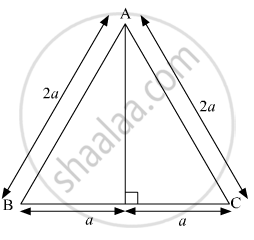Share

# Solve the Following Example. Find the Height of an Equilateral Triangle Having Side 2a. - Geometry

ConceptApollonius Theorem

#### Question

Solve the following example.

Find the height of an equilateral triangle having side 2a.

#### SolutionSince, ABC is an equilateral triangle, AD is the perpendicular bisector of BC.
Now, According to Pythagoras theorem,
In ∆ABD

${AB}^2 = {AD}^2 + {BD}^2$
$\Rightarrow \left( 2a \right)^2 = {AD}^2 + a^2$
$\Rightarrow 4 a^2 - a^2 = {AD}^2$
$\Rightarrow {AD}^2 = 3 a^2$
$\Rightarrow AD = \sqrt{3}a$

Hence, the height of an equilateral triangle is

$\sqrt{3}a$
Is there an error in this question or solution?

#### APPEARS IN

Solution Solve the Following Example. Find the Height of an Equilateral Triangle Having Side 2a. Concept: Apollonius Theorem.
S